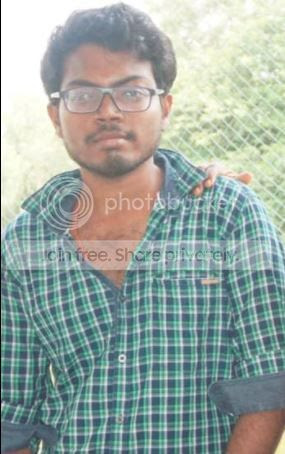# Pharma Engineering

For Engineer By Engineer

• ## Saturday, 21 November 2020

Hope everyone is doing well.

Received a query requesting the detailed design of agitators instead of general design which i've provided earlier.

Today i'm gonna provide some detailed technical notes on designing of pitch blade turbine.

Most of the design equations were derived based on the equipment's GA Drawing and some were gathered from my experience. Let's hope these will help you.

Before that we gonna discuss some key points and significance of using a PBT (Pitch Blade Turbine).

What are the cases to use PBT ?

For less dense and less viscous reaction mass we prefer PBT, the mixing with PBT shall be axial, which will allow the mass to pump from bottom to top uniformly.

Will PBT generate top pumping or bottom pumping or both ?

PBT will generate top pumping i.e., lifting mass from bottom to top.

Lets take an example of 100 L SS Reactor with PBT agitator design, in general we consider 80% occupancy for a reactor. Water as medium. Basic dimensions: Internal Dia - 48 cm, Height - 58 cm, Shell thickness - 0.6 cm.

Let's start the design [majority of the design calculations were of thumb rules],

Dish height = 0.2 x inner dia = 0.2 x 48 = 9.6 cm,

Impeller Dia = 40 % sweep = 0.4 x 58 = 19.2 cm,

Blade width = Impeller Dia / 5 = 19.2 / 5 = 3.84 cm,

Blade thickness = Vessel thickness / 2 = 0.6 / 2 = 0.3 cm,

Liquid level = ((((1000 x liquid vol.)-(0.0809 x Inner dia^3))x4) / (3.141 x Inner dia^2)) + Dish heigh  = 48.9 cm

Impeller count = Liquid level x density / impeller dia = 48.89 x 1 / 19.2 = 2.54 ~3

Distance between impeller = Impeller Dia = 19.2 cm,

Volume of reactor = (((3.141 x Inner dia^2 x Vessel height)/4)+(0.0809 x Inner dia^3))/1000 = 113.9 Lts,

Tip Speed = 3.141 x 19.2 x 140 / 60 x 100 = 1.41 m/sec,

Distance between reactor bottom and impeller = Liquid height / 7 = 48.9 / 7 = 6.99 cm,

Liquid density = 1000 Kg/m3,

Liquid viscosity = 0.0008 Kg-m/sec,

Reynolds number = RPS x impeller dia ^2 x density / viscosity = 107365,

Power number = 2.5 [As per generic agitator curves],

Power req. for agitation = Np x density x Impeller Dia^5 x RPS^3 = 8.24 W = 0.011 HP,

Transmission losses = 20%,
Gland losses = 10%,
Gear box losses = 10%,
Agitation power required after considering losses = 0.011 x (20+10+10)/100 = 0.015 HP,

Power with 70% efficiency, i.e., 0.015 / 0.7 = 0.022 HP,

/** Mixing dynamics calculation shall be updated in a short time **/

Baffles Design:

Number of baffles = 4 (say)

Baffle width = Internal dia / 12 = 4 cm,

Baffle height = Vessel height x 0.8 = 46.4 cm,

Baffle thickness = 0.5 - 1 cm,

Bottom clearance = Baffle width / 5 = 0.8 cm,

Distance between baffle and shell = Inner dia / 24 = 2 cm.

That's it ....!!!

These are some of the aspects related to design of PBT agitator.

If any queries, feel free to comment / message me ......!!!

Is the design helpful and a simple reference ?
Yes
No
Not really
Seems critical

A
bout The AuthorHi! I am Ajay Kumar Kalva, Currently serving as the CEO of this site, a tech geek by passion, and a chemical process engineer by profession, i'm interested in writing articles regarding technology, hacking and pharma technology.Hi! I am Ajay Kumar Kalva, Currently serving as the CEO of this site, a tech geek by passion, and a chemical process engineer by profession, i'm interested in writing articles regarding technology, hacking and pharma technology.